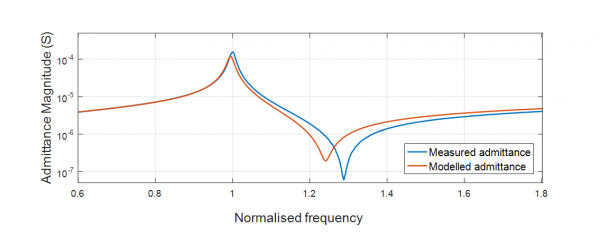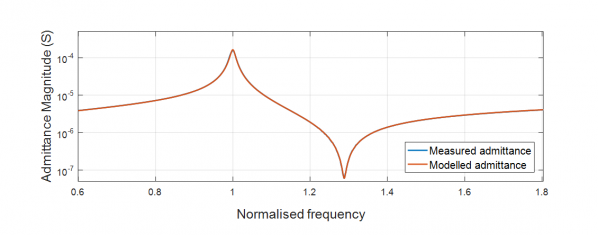Reducing SONAR overheating

31 October 2017

Blogger: Marcus Wild, PhD-candidate, Centre for Innovative Ultrasound Solutions (CIUS) and the University College of Southeast Norway.

Within the maritime industry, ships use SONAR (Sound Navigation and Ranging) systems in order to make maps of the seabed. The problem with SONARs, is that they have a tendency to overheat. Our aim is to predict the temperature rise in the materials used in SONARs to prevent this from happening.

SONARs emit sound waves, which then bounce off the seabed and return to the ship, in the same way that a bat or a dolphin uses sound to find its prey. SONARs are made of many components, but one of the most important components is the piezoelectric (piezo means squeeze) material. When pressure is applied to this type of material, an electric current is produced and reversely, when an electric current is applied to this material, it generates a sound wave (like a microphone and an amplifier). This behaviour is very useful and this material is the driving mechanism behind the sound wave generated by SONAR. However, some of this energy is also converted into heat, and too much heat will damage the SONAR.

In order to predict the heating in the piezoelectric material, the material properties such as the stiffness, electric properties (specifically, electric permittivity) and the electromechanical coupling factor (how easily the material converts mechanical energy like sound to electrical energy and vice versa) need to be characterised accurately.

First, we developed a fast and accurate method to characterize the piezoelectric material. This method uses a 1D analytical model of a piezoelectric material, which predicts the material’s electrical admittance as a function of frequency for a given set of material parameters. The electric admittance is the ease that a material has to allow an electrical current to flow through it for a given voltage. This property is frequency dependent for a piezoelectric material. The shape of the electrical admittance as a function of frequency can give us a lot of information about the material properties such as the resonance frequency or the energy loss mechanisms. The electrical admittance can also be measured quite easily in our case.

Inevitably, there will be a difference between the actual and modelled electrical admittance. To reduce the difference between theory and practice, we use an optimisation or minimisation algorithm. This algorithm will feed the 1D model with a series of changing material properties, which produces a different admittance curve for each change. It will do this until it finds a set of material parameters where the model mirrors reality. At that point, we have characterised the material in question accurately.

The efficacy of the method is illustrated in figure 1 and 2. Figure 1 shows that there is a clear difference between the two curves before optimisation. This suggests that the initial material parameters input into the 1D model are not accurate. After the optimisation however, there is no discernible difference between the two curves as shown in figure 2, and the material parameter set is the accurate set for this material.Figure 1: Comparison between measured and modelled admittance curve as a function of frequency before optimisation.Figure 2: Comparison between measured and modelled admittance curve as a function of frequency after optimisation.

Now that we have developed a method to characterise the piezoelectric material, we will apply it to the piezoelectric material at various temperatures. Indeed, the material parameters tend to change with temperature, and this is important for us to capture if we are to predict the heat rise within this material. Once we have these material parameters, we will be able to predict the amount of energy lost by this material depending on time and volume. This will enable us to be a step closer to understanding how and where the heat is generated in these materials.

0